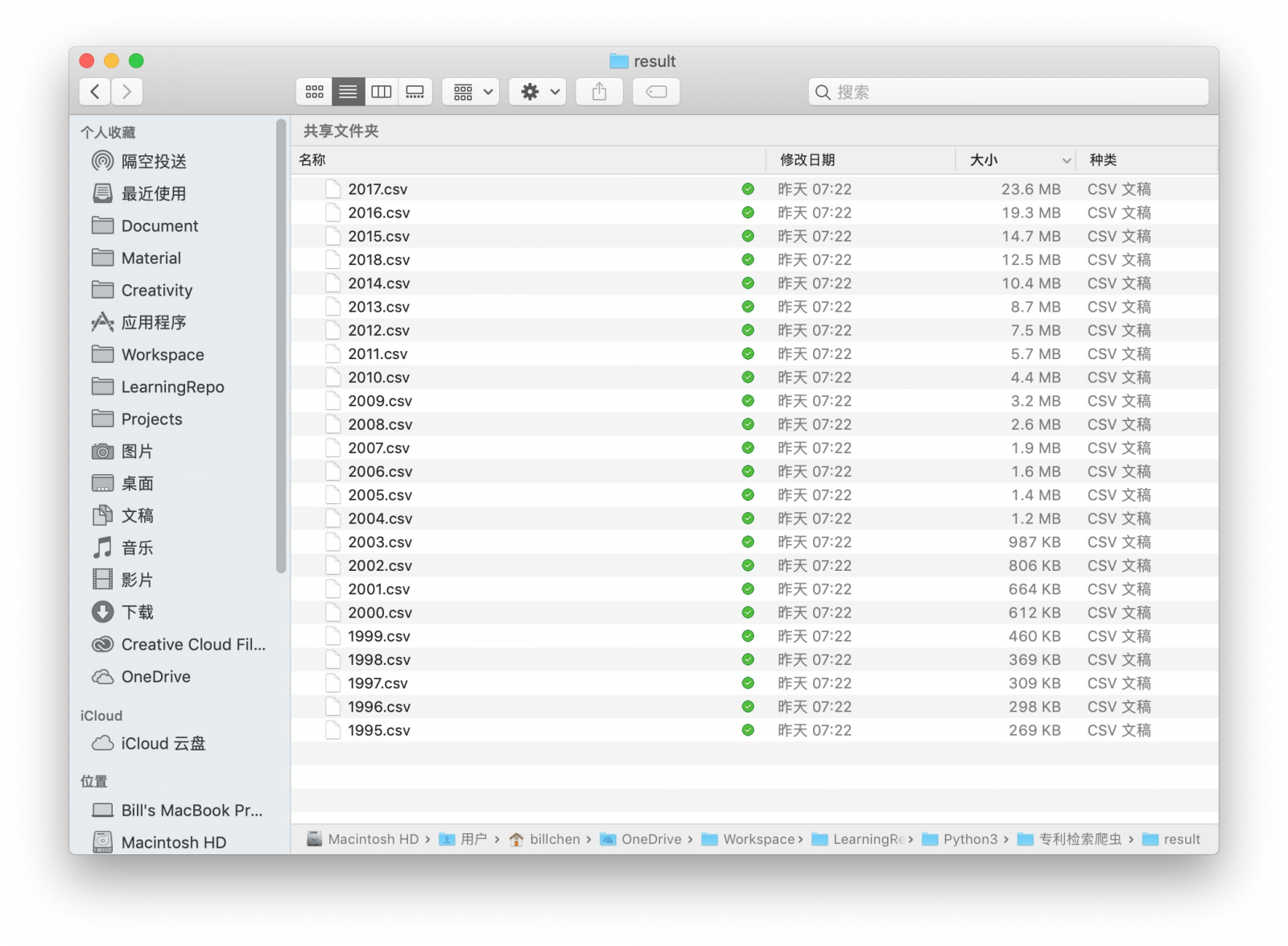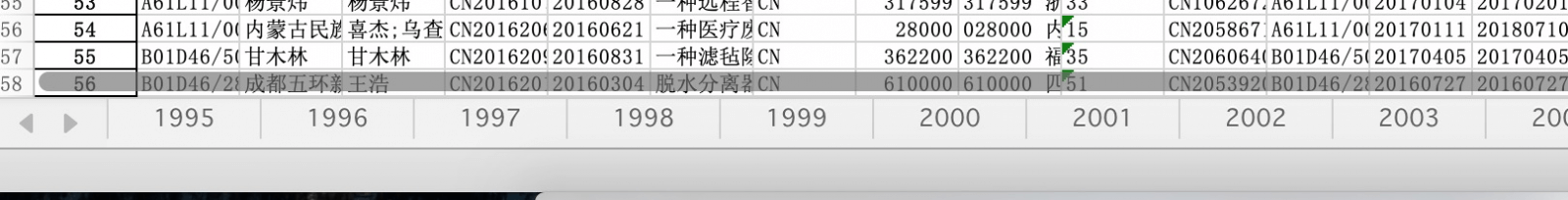``````while year <= 2018:
csvPath = sys.path + '/result/%d.csv' % year
excelPath = sys.path + '/result.xlsx'
excelWriter = pandas.ExcelWriter(excelPath)
print("正在将 %d 年的 %d 条数据转换为 xlsx..." % (year, countThis))
year = year + 1
``````

``````# 依赖 openpyxl 库
from openpyxl import load_workbook

while year <= 2018:
csvPath = sys.path + '/result/%d.csv' % year
excelPath = sys.path + '/result.xlsx'
# 增加 engine='openpyxl' 一栏
excelWriter = pandas.ExcelWriter(excelPath, engine='openpyxl'）
# 使用 openpyxl 来把现有数据传递给excelWriter，使其在写入的时候保留原本数据